# Mathematics | Walks, Trails, Paths, Cycles and Circuits in Graph

Prerequisite – Graph Theory Basics – Set 1
1. Walk –
A walk is a sequence of vertices and edges of a graph i.e. if we traverse a graph then we get a walk.
Vertex can be repeated
Edges can be repeated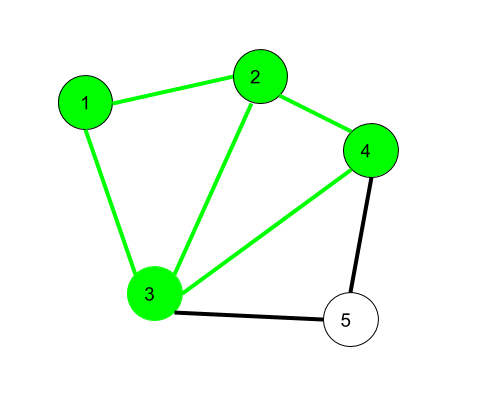Here 1->2->3->4->2->1->3 is a walk

```Walk can be open or closed.
Walk can be repeated anything (edges or vertices).```

2. Trail –
A Walk in which no edge is repeated then we get a trail
Vertex can be repeated
Edges not repeated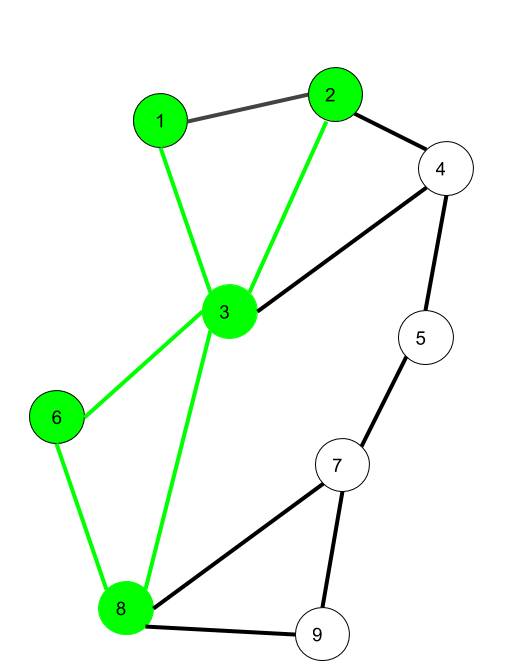Here 1->3->8->6->3->2 is trail
Also 1->3->8->6->3->2->1 will be a closed trail

3. Circuit –
Traversing a graph such that not an edge is repeated but vertex can be repeated and it is closed also i.e. it is a closed trail.
Vertex can be repeated
Edge not repeated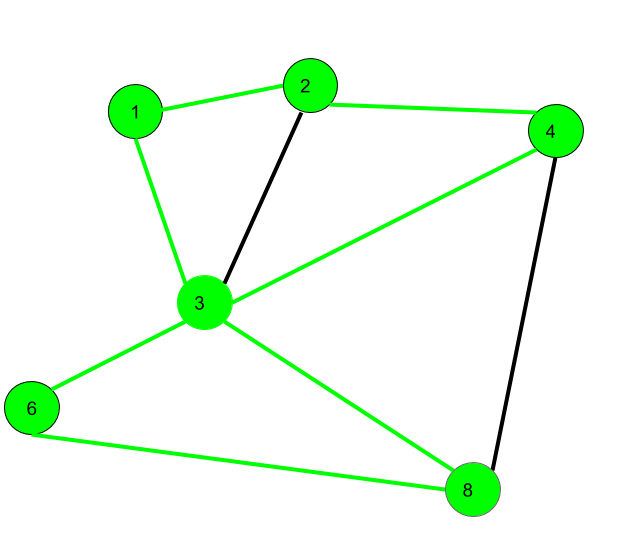Here 1->2->4->3->6->8->3->1 is a circuit

```Circuit is a closed trail.
These can have repeated vertices only.```

4. Path –
It is a trail in which neither vertices nor edges are repeated i.e. if we traverse a graph such that we do not repeat a vertex and nor we repeat an edge.
Vertex not repeated
Edge not repeated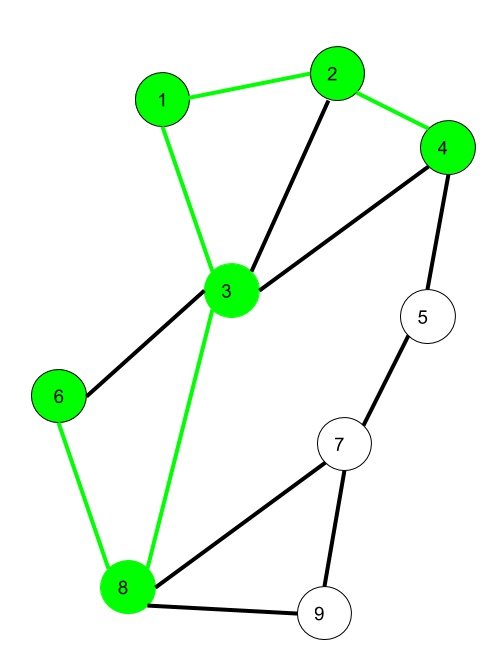Here 6->8->3->1->2->4 is a Path

5. Cycle –
Traversing a graph such that we do not repeat a vertex nor we repeat a edge but the starting and ending vertex must be same i.e. we can repeat starting and ending vertex only then we get a cycle.
Vertex not repeated
Edge not repeatedHere 1->2->4->3->1 is a cycle.

```Cycle is a closed path.
These can not have repeat anything (neither edges nor vertices).```

Note that for closed sequences start and end vertices are the only ones that can repeat.My Personal Notes arrow_drop_up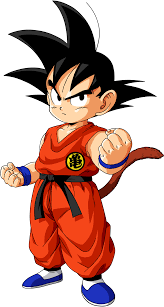If you like GeeksforGeeks and would like to contribute, you can also write an article using contribute.geeksforgeeks.org or mail your article to contribute@geeksforgeeks.org. See your article appearing on the GeeksforGeeks main page and help other Geeks.

Please Improve this article if you find anything incorrect by clicking on the "Improve Article" button below.